Ch 6. Integrals Multimedia Engineering Math IndefiniteIntegral Area DefiniteIntegral FundamentalTheorem SubstitutionRule
 Chapter 1. Limits 2. Derivatives I 3. Derivatives II 4. Mean Value 5. Curve Sketching 6. Integrals 7. Inverse Functions 8. Integration Tech. 9. Integrate App. 10. Parametric Eqs. 11. Polar Coord. 12. Series Appendix Basic Math Units Search eBooks Dynamics Fluids Math Mechanics Statics Thermodynamics Author(s): Hengzhong Wen Chean Chin Ngo Meirong Huang Kurt Gramoll ©Kurt GramollMATHEMATICS - THEORY In indefinite integral section, various formulas have been introduced to calculate the integral of a function. However, it is not possible to have a formula for every possible situation. Thus, basic formulas like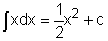need to be generalized so they can be used in a variety of cases. This rule is called the substitution rule. The Substitution Rule for Indefinite Integrals The substitution rule states: If u = g(x) is a differentiable function in an interval R and f is continuous on R, then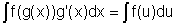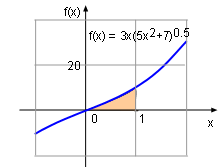Original Function f(x)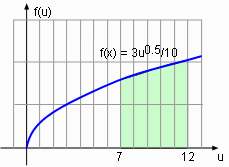Substitution Function for f(x) This rule can be better understand by calculating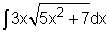. Let u = 5x2 + 7. The derivative of u with respect to x is      du/dx = 10x Rearrange the above equation gives      du = 10xdx Thus ĀĀĀĀĀ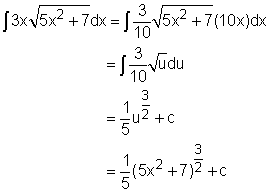In order to double check the answer, the derivative of function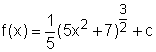can be taken.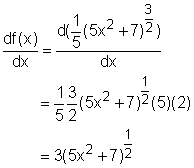The result confirms the correction of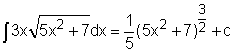. The Substitution Rule for Definite Integrals Substitution rule can also be used for definite integrals. The substitution rule for definite integrals states: If g'(x) is continuous on interval R and f(x) is continuous on the range of g(x), then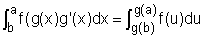This rule can be better understand by calculating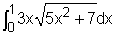. Let u = 5x2 + 7. The derivative of u with respect to x is      du/dx = 10x Rearrange the above equation gives      du = 10xdx The new limits of integration can be calculated. When x = 0, u = 7 and when x = 1, u = 12. Therefore,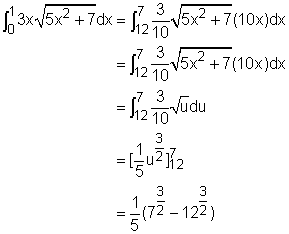It is obviously that the substitution rule provides an easy way to calculate the integral of a function.